login  home  contents  what's new  discussion  bug reports help  links  subscribe  changes  refresh  edit

## Reference

Matolcsi 4.2.3 op. cit.
In contradistinction to the non-relativistic case and to our habitual "evidence", the relative velocity of P with respect to Q is not the opposite of the relative velocity of Q with respect to P ... It is worth emphasizing this fact because in most textbooks one takes it for granted that w(P,Q) and -w(Q,P) are equal: "If an observer moves with velocity v relative to another then the second observer moves with velocity -v relative to the first one.". Nevertheless, no harm comes because vectors are given there by their components with respect to some convenient bases and then the components of w(P,Q) and w(Q,P) become opposite to each other.

## Minkowski Package

This code extends the Minkowski package defined on the previous page SandBox Lorentz Transformations

fricas
(1) -> <aldor>
#include "axiom.as"
#pile
#library minkowski1 "mink1.ao"
EXPR     ==> Expression
FRAC     ==> Fraction
INT      ==> Integer
LIST     ==> List
MATRIX   ==> Matrix
PI       ==> PositiveInteger
NNI      ==> NonNegativeInteger
SQMATRIX ==> SquareMatrix
import from INT, NNI
import from MATRIX EXPR INT
import from EXPR INT
import from SQMATRIX(4,EXPR INT)
import from FRAC INT
import from LIST EXPR INT
minkowski2(): with
M: (MATRIX EXPR INT,MATRIX EXPR INT) -> MATRIX EXPR INT
L: SQMATRIX(4,EXPR INT)              -> SQMATRIX(4,EXPR INT)
import from minkowski1
inline from minkowski1
-- Something wrong with the import so these defined locally
g():Matrix EXPR INT == diagonalMatrix([-1,1,1,1])$Matrix(EXPR INT) g(x:Matrix EXPR INT):Matrix EXPR INT == transpose(x)*g() -- Boost Bivector M(P:MATRIX EXPR INT,Q:MATRIX EXPR INT):MATRIX EXPR INT == (P*g(Q) - Q*g(P)) -- Lorentz Transformation L(b:SQMATRIX(4,EXPR INT)):SQMATRIX(4,EXPR INT) == id := 1$SQMATRIX(4,EXPR INT)
lambda:EXPR(INT) := 1/(1+sqrt(1+1/2*trace(b^2)))
id + b + lambda * b^2</aldor>
fricas
Compiling FriCAS source code from file
/var/lib/zope2.10/instance/axiom-wiki/var/LatexWiki/mink2.as
using Aldor compiler and options
-O -Fasy -Fao -Flsp -lfricas -Mno-ALDOR_W_WillObsolete -DFriCAS -Y $FRICAS/algebra -I$FRICAS/algebra
Use the system command )set compiler args to change these
options.
The )library system command was not called after compilation.

fricas
)lib mink1
fricas
Reading /var/aw/var/LatexWiki/mink1.asy
minkowski1 is now explicitly exposed in frame initial
minkowski1 will be automatically loaded when needed from
/var/aw/var/LatexWiki/mink1
fricas
)lib mink2
fricas
Reading /var/aw/var/LatexWiki/mink2.asy
minkowski2 is now explicitly exposed in frame initial
minkowski2 will be automatically loaded when needed from
/var/aw/var/LatexWiki/mink2

Objects and velocities

fricas
P:=obs();
>> System error:
#<SB-SYS:FD-STREAM for "file /var/aw/var/LatexWiki/mink1.fasl" {1004BECB53}> is
a fasl file compiled with SBCL 1.0.31, and can't be loaded into SBCL 1.1.1.

Choose three arbitrary basis vectors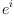in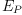(i.e such that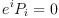)

fricas
e:=matrix [[e1,e2,e3],_
[ 1, 0, 0],_
[ 0, 1, 0],_
[ 0, 0, 1]]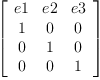(1)
Type: Matrix(Polynomial(Integer))
fricas
e:=eval(e,solve(map(x+->(x=0),members(g(P)*e)),[e1,e2,e3]).1)
There are 1 exposed and 0 unexposed library operations named g
having 1 argument(s) but none was determined to be applicable.
Use HyperDoc Browse, or issue
)display op g
package-calling the operation or using coercions on the arguments
will allow you to apply the operation.
Cannot find a definition or applicable library operation named g
with argument type(s)
Variable(P)
Perhaps you should use "@" to indicate the required return type,
or "$" to specify which version of the function you need. Express the coefficients of relative velocity u in this basis fricas u!:=matrix [[u1],[u2],[u3]]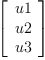(2) Type: Matrix(Polynomial(Integer)) fricas u!:=eval(u!,solve(map(x+->(x=0),members(u-e*u!)),[u1,u2,u3]).1) There are 9 exposed and 6 unexposed library operations named - having 2 argument(s) but none was determined to be applicable. Use HyperDoc Browse, or issue )display op - to learn more about the available operations. Perhaps package-calling the operation or using coercions on the arguments will allow you to apply the operation. Cannot find a definition or applicable library operation named - with argument type(s) Variable(u) Matrix(Polynomial(Integer)) Perhaps you should use "@" to indicate the required return type, or "$" to specify which version of the function you need.

The Lorentz transformation transforms the basis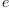to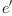in such a way that the components of the inverse velocity with respect to basisare just minus (-) the components of the velocity with respect to the basis.

fricas
e' := L(M(P,Q))*e;
There are 1 exposed and 0 unexposed library operations named M
having 2 argument(s) but none was determined to be applicable.
Use HyperDoc Browse, or issue
)display op M
package-calling the operation or using coercions on the arguments
will allow you to apply the operation.
Cannot find a definition or applicable library operation named M
with argument type(s)
Variable(P)
Variable(Q)
Perhaps you should use "@" to indicate the required return type,
or "$" to specify which version of the function you need. ## Composition of collinear velocities (again) For velocity v collinear with reciprocal velocity u' we can use Einstein's original formula. Reference: Matolcsi 4.3.4. op. cit. See SandBoxCategoricalRelativity. Find object L such that v=w(Q,L) where fricas v := alpha*u'; Type: Polynomial(Integer) fricas L := b(Q,v); There are 1 exposed and 0 unexposed library operations named b having 2 argument(s) but none was determined to be applicable. Use HyperDoc Browse, or issue )display op b to learn more about the available operations. Perhaps package-calling the operation or using coercions on the arguments will allow you to apply the operation. Cannot find a definition or applicable library operation named b with argument type(s) Variable(Q) Polynomial(Integer) Perhaps you should use "@" to indicate the required return type, or "$" to specify which version of the function you need.

First express the coefficients of the composite relative velocity w(P,L) and the relative velocity v=w(Q,L) in the basesandfricas
w!:=matrix [[w1],[w2],[w3]];
Type: Matrix(Polynomial(Integer))
fricas
w!:=eval(w!,solve(map(x+->(x=0),members(w(P,L)-e*w!)),[w1,w2,w3]).1);
There are 1 exposed and 0 unexposed library operations named w
having 2 argument(s) but none was determined to be applicable.
Use HyperDoc Browse, or issue
)display op w
package-calling the operation or using coercions on the arguments
will allow you to apply the operation.
Cannot find a definition or applicable library operation named w
with argument type(s)
Variable(P)
Variable(L)
Perhaps you should use "@" to indicate the required return type,
or "$" to specify which version of the function you need. Then in Einstein's equation we add u! and v! even though they have different bases: fricas abs(u)==sqrt((transpose(u)*u).(1,1)) Type: Void fricas Is?( w!=(u!+v!)/(1+abs(u!)*abs(v!)) ) There are 13 exposed and 11 unexposed library operations named + having 2 argument(s) but none was determined to be applicable. Use HyperDoc Browse, or issue )display op + to learn more about the available operations. Perhaps package-calling the operation or using coercions on the arguments will allow you to apply the operation. Cannot find a definition or applicable library operation named + with argument type(s) Matrix(Polynomial(Integer)) Variable(v!) Perhaps you should use "@" to indicate the required return type, or "$" to specify which version of the function you need.

 Subject:   Be Bold !! ( 14 subscribers )